JMSLTM Numerical Library 7.2.0
com.imsl.stat

## Class Ranks

• ```public class Ranks
extends Object```
Compute the ranks, normal scores, or exponential scores for a vector of observations.

The class Ranks can be used to compute the ranks, normal scores, or exponential scores of the data in X. Ties in the data can be resolved in four different ways, as specified by member function `setTieBreaker`. The type of values returned can vary depending on the member function called:

GetRanks: Ordinary Ranks

For this member function, the values output are the ordinary ranks of the data in X. If X[i] has the smallest value among those in X and there is no other element in X with this value, then `getRanks(i)` = 1. If both X[i] and X[j] have the same smallest value, then

if TieBreaker = 0, Ranks[i] = `getRanks([j]` = 1.5
if TieBreaker = 1, Ranks[i] = Ranks[j] = 2.0
if TieBreaker = 2, Ranks[i] = Ranks[j] = 1.0
if TieBreaker = 3, Ranks[i] = 1.0 and Ranks[j] = 2.0
or Ranks[i] = 2.0 and Ranks[j] = 1.0.

When the ties are resolved by use of function `setRandom`, different results may occur when running the same program at different times unless the "seed" of the random number generator is set explicitly by use of `Random` method `setSeed`. Ordinarily, there is no need to call the routine to set the seed, even if there are ties in the data.

getBlomScores: Normal Scores, Blom Version

Normal scores are expected values, or approximations to the expected values, of order statistics from a normal distribution. The simplest approximations are obtained by evaluating the inverse cumulative normal distribution function, `inverseNormal`, at the ranks scaled into the open interval (0, 1). In the Blom version (see Blom 1958), the scaling transformation for the rank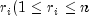, where n is the sample size is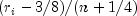. The Blom normal score corresponding to the observation with rank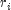is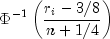where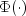is the normal cumulative distribution function.

Adjustments for ties are made after the normal score transformation. That is, if X[i] equals X[j] (within fuzz) and their value is the k-th smallest in the data set, the Blom normal scores are determined for ranks of k and k + 1, and then these normal scores are averaged or selected in the manner specified by TieBreaker, which is set by the method `setTieBreaker`. (Whether the transformations are made first or ties are resolved first makes no difference except when averaging is done.)

getTukeyScores: Normal Scores, Tukey Version

In the Tukey version (see Tukey 1962), the scaling transformation for the rankis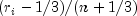. The Tukey normal score corresponding to the observation with rankis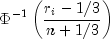Ties are handled in the same way as discussed above for the Blom normal scores.

getVanDerWaerdenScores: Normal Scores, Van der Waerden Version

In the Van der Waerden version (see Lehmann 1975, page 97), the scaling transformation for the rankis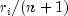. The Van der Waerden normal score corresponding to the observation with rankis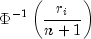Ties are handled in the same way as discussed above for the Blom normal scores.

getNormalScores: Expected Value of Normal Order Statistics

The method `getNormalScores` returns the expected values of the normal order statistics. If the value in X[i] is the k-th smallest, then the value `getNormalScores[i]` is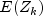, where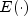is the expectation operator and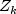is the k-th order statistic in a sample of size `NOBS` from a standard normal distribution. Ties are handled in the same way as discussed above for the Blom normal scores.

getSavageScores: Savage Scores

The method `getSavageScores` returns the expected values of the exponential order statistics. These values are called Savage scores because of their use in a test discussed by Savage (1956) (see Lehman 1975). If the value in X[i] is the k-th smallest, then the i-th output value output is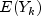, where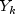is the k-th order statistic in a sample of size n from a standard exponential distribution. The expected value of the k-th order statistic from an exponential sample of size n is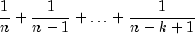Ties are handled in the same way as discussed above for the Blom normal scores.

Example
• ### Field Summary

Fields
Modifier and Type Field and Description
`static int` `TIE_AVERAGE`
In case of ties, use the average of the scores of the tied observations.
`static int` `TIE_HIGHEST`
In case of ties, use the highest score in the group of ties.
`static int` `TIE_LOWEST`
In case of ties, use the lowest score in the group of ties.
`static int` `TIE_RANDOM`
In case of ties, use one of the group of ties chosen at random.
• ### Constructor Summary

Constructors
Constructor and Description
`Ranks()`
Constructor for the Ranks class.
• ### Method Summary

Methods
Modifier and Type Method and Description
`static double` ```expectedNormalOrderStatistic(int i, int n)```
Returns the expected value of a normal order statistic.
`double[]` `getBlomScores(double[] x)`
Gets the Blom version of normal scores for each observation.
`double[]` `getNormalScores(double[] x)`
Gets the expected value of normal order statistics (for tied observations, the average of the expected normal scores).
`double[]` `getRanks(double[] x)`
Gets the rank for each observation.
`double[]` `getSavageScores(double[] x)`
Gets the Savage scores (the expected value of exponential order statistics).
`double[]` `getTukeyScores(double[] x)`
Gets the Tukey version of normal scores for each observation.
`double[]` `getVanDerWaerdenScores(double[] x)`
Gets the Van der Waerden version of normal scores for each observation.
`void` `setFuzz(double fuzz)`
Sets the fuzz factor used in determining ties.
`void` `setRandom(Random random)`
Sets the `Random` object.
`void` `setTieBreaker(int iTie)`
Sets the tie breaker for Ranks.
• ### Methods inherited from class java.lang.Object

`clone, equals, finalize, getClass, hashCode, notify, notifyAll, toString, wait, wait, wait`
• ### Field Detail

• #### TIE_AVERAGE

`public static final int TIE_AVERAGE`
In case of ties, use the average of the scores of the tied observations.
Constant Field Values
• #### TIE_HIGHEST

`public static final int TIE_HIGHEST`
In case of ties, use the highest score in the group of ties.
Constant Field Values
• #### TIE_LOWEST

`public static final int TIE_LOWEST`
In case of ties, use the lowest score in the group of ties.
Constant Field Values
• #### TIE_RANDOM

`public static final int TIE_RANDOM`
In case of ties, use one of the group of ties chosen at random.
Constant Field Values
• ### Constructor Detail

• #### Ranks

`public Ranks()`
Constructor for the Ranks class.
• ### Method Detail

• #### expectedNormalOrderStatistic

```public static double expectedNormalOrderStatistic(int i,
int n)```
Returns the expected value of a normal order statistic.
Parameters:
`i` - an `int`, the rank of the order statistic
`n` - an `int`, the sample size
Returns:
a `double`, the expected value of the i-th order statistic in a sample of size n from the standard normal distribution
• #### getBlomScores

`public double[] getBlomScores(double[] x)`
Gets the Blom version of normal scores for each observation.
Parameters:
`x` - a `double` array which contains the observations to be ranked
Returns:
a `double` array which contains the Blom version of normal scores for each observation in x
• #### getNormalScores

`public double[] getNormalScores(double[] x)`
Gets the expected value of normal order statistics (for tied observations, the average of the expected normal scores).
Parameters:
`x` - a `double` array which contains the observations
Returns:
a `double` array which contains the expected value of normal order statistics for the observations in x (for tied observations, the average of the expected normal scores)
• #### getRanks

`public double[] getRanks(double[] x)`
Gets the rank for each observation.
Parameters:
`x` - a `double` array which contains the observations to be ranked
Returns:
a `double` array which contains the rank for each observation in x
• #### getSavageScores

`public double[] getSavageScores(double[] x)`
Gets the Savage scores (the expected value of exponential order statistics).
Parameters:
`x` - a `double` array which contains the observations
Returns:
a `double` array which contains the Savage scores for the observations in x. (the expected value of exponential order statistics)
• #### getTukeyScores

`public double[] getTukeyScores(double[] x)`
Gets the Tukey version of normal scores for each observation.
Parameters:
`x` - a `double` array which contains the observations to be ranked
Returns:
a `double` array which contains the Tukey version of normal scores for each observation in x
• #### getVanDerWaerdenScores

`public double[] getVanDerWaerdenScores(double[] x)`
Gets the Van der Waerden version of normal scores for each observation.
Parameters:
`x` - a `double` array which contains the observations to be ranked
Returns:
a `double` array which contains the Van der Waerden version of normal scores for each observation in x
• #### setFuzz

`public void setFuzz(double fuzz)`
Sets the fuzz factor used in determining ties.
Parameters:
`fuzz` - a `double` which represents the fuzz factor
• #### setRandom

`public void setRandom(Random random)`
Sets the `Random` object.
Parameters:
`random` - a `Random` object used in breaking ties
• #### setTieBreaker

`public void setTieBreaker(int iTie)`
Sets the tie breaker for Ranks.
Parameters:
`iTie` - an `int` which represents the tie breaker
JMSLTM Numerical Library 7.2.0